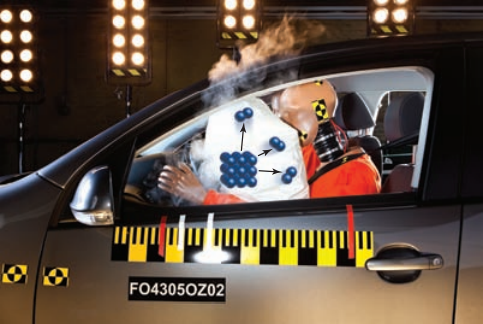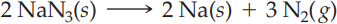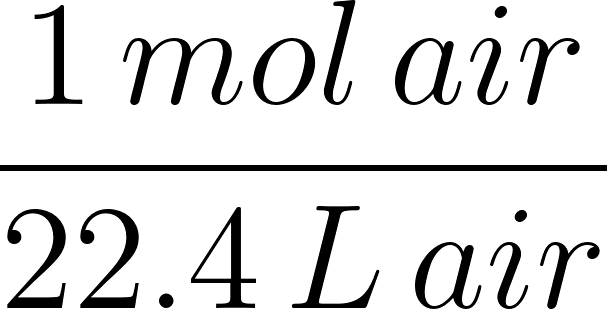×
Get Full Access to Introductory Chemistry - 5 Edition - Chapter 11 - Problem 125p
Get Full Access to Introductory Chemistry - 5 Edition - Chapter 11 - Problem 125p

×

# Automobile air bag inflate following a serious impact. The impact triggers the chemicalISBN: 9780321910295 34

## Solution for problem 125P Chapter 11

Introductory Chemistry | 5th Edition

• Textbook Solutions
• 2901 Step-by-step solutions solved by professors and subject experts
• Get 24/7 help from StudySoup virtual teaching assistantsIntroductory Chemistry | 5th Edition

4 5 1 295 Reviews
12
0
Problem 125P

Automobile air bag inflate following a serious impact. The impact triggers the chemical reaction:

2 NaN3(s) ⟶ 2 Na(s) + 3 N2( g)

If an automobile air bag has a volume of 11.8 L, how much NaN3 in grams is required to fully inflate the air bag upon impact? Assume STP conditions.Step-by-Step Solution:

Problem 125P

Automobile air bag inflate following a serious impact. The impact triggers the chemical reaction:If an automobile air bag has a volume of 11.8 L, how much NaN3 in grams is required to fully inflate the air bag upon impact? Assume STP conditions.

Step by Step Solution

Step 1 of 3

From the given,

Initial volume of air bag = 11.8 L

1 mole = 22.4L

The conversion factor ;Let’s calculate the number of moles of air present in 11.8 L of air bag.Step 2 of 3

Step 3 of 3

##### ISBN: 9780321910295

The full step-by-step solution to problem: 125P from chapter: 11 was answered by , our top Chemistry solution expert on 05/06/17, 06:45PM. This textbook survival guide was created for the textbook: Introductory Chemistry, edition: 5. The answer to “?Automobile air bag inflate following a serious impact. The impact triggers the chemical reaction: 2 NaN3(s) ? 2 Na(s) + 3 N2( g) If an automobile air bag has a volume of 11.8 L, how much NaN3 in grams is required to fully inflate the air bag upon impact? Assume STP conditions.” is broken down into a number of easy to follow steps, and 52 words. This full solution covers the following key subjects: air, bag, impact, nan, automobile. This expansive textbook survival guide covers 19 chapters, and 2046 solutions. Introductory Chemistry was written by and is associated to the ISBN: 9780321910295. Since the solution to 125P from 11 chapter was answered, more than 351 students have viewed the full step-by-step answer.

Unlock Textbook Solution